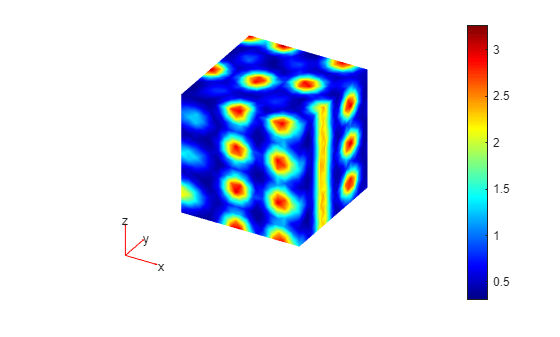# Nonlinear System with Cross-Coupling Between Components

This example shows how to solve a nonlinear PDE system of two equations with cross-coupling between the two components. The system is a Schnakenberg system

`$\begin{array}{l}\frac{\partial {\mathit{u}}_{1}}{\partial \mathit{t}}-{\mathit{D}}_{1}\Delta {\mathit{u}}_{1}=\kappa \left(\mathit{a}-{\mathit{u}}_{1}+{{\mathit{u}}_{1}}^{2}{\mathit{u}}_{2}\right)\\ \frac{\partial {\mathit{u}}_{2}}{\partial \mathit{t}}-{\mathit{D}}_{2}\Delta {\mathit{u}}_{2}=\kappa \left(\mathit{b}-{{\mathit{u}}_{1}}^{2}{\mathit{u}}_{2}\right)\end{array}$`

with the steady-state solution ${\mathit{u}}_{1\mathit{S}}=\mathit{a}+\mathit{b}$ and ${\mathit{u}}_{2\mathit{S}}=\frac{\mathit{b}}{{\left(\mathit{a}+\mathit{b}\right)}^{2}}$. The initial conditions are a small perturbation of the steady-state solution.

### Solution for First Time Span

First, create a PDE model for a system of two equations.

`model = createpde(2);`

Create a cubic geometry and assign it to the model.

```gm = multicuboid(1,1,1); model.Geometry = gm;```

Generate the mesh using the linear geometric order to save memory.

`generateMesh(model,'GeometricOrder','linear');`

Define the parameters of the system.

```D1 = 0.05; D2 = 1; kappa = 100; a = 0.2; b = 0.8;```

Based on these parameters, specify the PDE coefficients in the toolbox format.

```d = [1;1]; c = [D1;D2]; f = @(region,state) [kappa*(a - state.u(1,:) + ... state.u(1,:).^2.*state.u(2,:)); kappa*(b - state.u(1,:).^2.*state.u(2,:)) ]; specifyCoefficients(model,'m',0,'d',d,'c',c,'a',0,'f',f);```

Set the initial conditions. The first component is a small perturbation of the steady-state solution ${\mathit{u}}_{1\mathit{S}}=\mathit{a}+\mathit{b}$. The second component is the steady-state solution ${\mathit{u}}_{2\mathit{S}}=\frac{\mathit{b}}{{\left(\mathit{a}+\mathit{b}\right)}^{2}}$.

```icFcn = @(region) [a + b + 10^(-3)*exp(-100*((region.x - 1/3).^2 ... + (region.y - 1/2).^2)); ... (b/(a + b)^2)*ones(size(region.x))]; setInitialConditions(model,icFcn);```

Solve the system for times 0 through 2 seconds.

```tlist = linspace(0,2,10); results = solvepde(model,tlist);```

Plot the first component of the solution at the last time step.

`pdeplot3D(model,'ColorMapData',results.NodalSolution(:,1,end));`### Initial Condition for Second Time Span Based on Previous Solution

Now, resume the analysis and solve the problem for times from 2 to 5 seconds. Reduce the magnitude of the previously obtained solution for time 2 seconds to 10% of the original value.

```u2 = results.NodalSolution(:,:,end); newResults = createPDEResults(model,u2(:)*0.1);```

Use `newResults` as the initial condition for further analysis.

`setInitialConditions(model,newResults);`

Solve the system for times 2 through 5 seconds.

```tlist = linspace(2,5,10); results25 = solvepde(model,tlist);```

Plot the first component of the solution at the last time step.

```figure pdeplot3D(model,'ColorMapData',results25.NodalSolution(:,1,end));```Alternatively, you can write a function that uses the results returned by the solver and computes the initial conditions based on the results of the previous analysis.

`NewIC = @(location) computeNewIC(results)`
```NewIC = function_handle with value: @(location)computeNewIC(results) ```

Remove the previous initial conditions.

`delete(model.InitialConditions);`

Set the initial conditions using the function `NewIC`.

`setInitialConditions(model,NewIC)`
```ans = GeometricInitialConditions with properties: RegionType: 'cell' RegionID: 1 InitialValue: @(location)computeNewIC(results) InitialDerivative: [] ```

Solve the system for times 2 through 5 seconds.

`results25f = solvepde(model,tlist);`

Plot the first component of the solution at the last time step.

```figure pdeplot3D(model,'ColorMapData',results25f.NodalSolution(:,1,end));```### Function Computing Initial Conditions

```function newU0 = computeNewIC(resultsObject) newU0 = 0.1*resultsObject.NodalSolution(:,:,end).'; end```